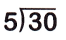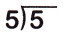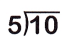# McGraw Hill Math Grade 3 Chapter 5 Lesson 4 Answer Key Dividing by 5

## McGraw-Hill Math Grade 3 Answer Key Chapter 5 Lesson 4 Dividing by 5

Solve

Look at each picture. Find the quotient.

Question 1.20 ÷ 5 = 4

Question 2.25 ÷ 5 = ___
25 ÷ 5 = 5

Explanation:
There are 25 apples
They are arranged as 5 rows of 5 apples each row
So, 25 ÷ 5 = 5.

Question 3.
0 ÷ 5 = ____
0 ÷ 5 = 0

Explanation:
Zero divided by any number is zero
So, 0 ÷ 5 = 0.

Divide. Use repeated subtraction to help.

Question 4.30 ÷ 5 = 6

Explanation:
I subtract 5 from 30 until i reach 0
I subtracted 5 from 30 six times
So, 30 ÷ 5 = 6.

Question 5.5 ÷ 5 = 1

Explanation:
Any number divided by itself is 1
So, 5 ÷ 5 = 1

Question 6.40 ÷ 5 = 8

Explanation:
I subtract 5 from 40 until i reach 0
I subtracted 5 from 40 eight times
So, 40 ÷ 5 = 8.

Question 7.